# Bootstrapping Approaches to Inference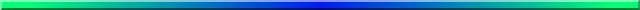The traditional parametric procedures that we all know and love are primarily based on several major assumptions about the population(s) from which our data came. For example, we almost routinely use procedures that assume that we are sampling from normal populations. (We can create tests based on other kinds of populations, but most tests assume normality. The point is that the test assumes something about the shape of the population, whether it be normal, exponential, logarithmic, or what-have-you.) We make such assumptions because they make our life easier. We know that if a distribution is normal, its mean and variance are independent. We know that if a distribution is normal, 95% of the observations will fall within 1.96 standard deviations from the mean. We know that if we sample from normal populations, the result of calculating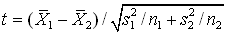will follow a Student's t distribution on n1 + n2 - 2 degrees of freedom. Knowing these things makes our life much easier, because we can easily draw conclusions about the data we have. For example, if we know that the mean of a set of difference scores is more than about two standard errors from 0.0, then we can conclude without further calculation that there has been a significant change from one measurement interval to another.

As nice as it is to be able to assume normality, and therefore know a great deal about our data before we even begin, there are problems. The most obvious problem is that we could be wrong. Perhaps our data are not remotely normally distributed. In that case, our inference may well be in error.

One of the very nice things statisticians have learned over the years is that, in many situations, the violation of the assumption of normality won't send us immediately to jail without passing "Go." Under a rather broad set of conditions we can violate our assumption and get away with it. By this I mean that our answer may still be correct even if our assumption is false. This is what we mean when we speak of a test as being robust.

However, this still leaves at least two problems. In the first place, it is not hard to create reasonable data that violate a normality (or homogeneity of variance) assumption and have "true" answers that are quite different from the answer we would get by making a normality assumption. In other words, we can't always get away with violating assumptions. Second, there are many situations where even with normality, we don't know enough about the statistic we are using to draw the appropriate inferences. For example, one of the first things students learn in statistics is that the standard error of the mean can be nicely estimated as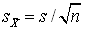. But what is the standard error of the median, or the standard error of the difference between medians? For the median we can come pretty close if we have normality. For the difference between medians, normality won't help us. We need some other way to find that standard error.

One way to look at bootstrap procedures is as procedures for handling data when we are not willing to make assumptions about the parameters of the populations from which we sampled. The most that we are willing to assume (and it is an absolutely critical assumption) is that the data we have are a reasonable representation of the population from which they came. We then resample from the pool of data that we have, and draw inferences about the corresponding population and its parameters.

The second way to look at bootstrap procedures is to think of them as what we use when we don't know enough. For example, if we don't know the standard error of the difference between medians, one thing we can do is to go ahead and draw many pairs of samples. For each pair we calculate, and record, the difference between the medians. Then the standard deviation of these differences is the standard error of the difference of medians. In other words, when we don't have an analytical (i.e. formula) solution, we use a brute-force empirical solution.

The basic idea behind bootstrapping is really very simple. The difficulties come when we try to deal with the niceties of the situation, and remove bias and/or instability. For right now I am going to ignore the niceties, but they are covered well in Efron and Tibshirani (1993), which is a classic in the field.

### The Bootstrap Conjecture

Gee, I've always wanted to be able to use an impressive phrase like that!

Imagine that we have a set of data drawn from some population. The elements of that population are X1, X2, X3, ...XN. This population has some parameter of interest (perhaps its median or variance), and we will call that θ. (People often get upset when mathematical types toss in generic terms labeled with Greek symbols. Well, sometimes we just have to do it. If it really bothers you, change θ to μ, or σ, or some other specific parameter you feel more comfortable with.) If we drew n observations from this population, calculated an estimate of θ, denoted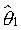, drew another n observations, calculated their estimate,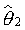, and so on, we could end up with the sampling distribution of θ. And the standard deviation of this distribution would be the standard error of θ. But to do this we either have to have the entire population at our fingertips, so that we can draw all of those samples, or we have to make an assumption, such as normality, so that we can compute what the standard error would be without drawing all of those samples. And if θ is some parameter that we don't have a formula for estimating, we have a problem even with normality.

Now suppose that we have a sample from that population. Denote the sample as x1, x2, ..., xn. (Notice that I have used lower case symbols to represent the values that I actually drew, whereas I used upper case symbols to represent the valuates in the population. That is a common device, and it is used as a way of keeping things clear.) The estimate of the parameter based on this sample is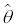. Now suppose that we treat the values x1, x2, ...xn as if they represented the population, and draw a sample of observations with replacement from these n values. For example, if n = 8, our sample might happen to contain x4, x2, x8, x2, x1, x2, x4, x5. Notice that, because we sampled with replacement, some values appeared more than once, and some values never appeared. For this sample we will calculate an estimate,*. I added the asterisk to indicate that this was an estimate based on a bootstrapped sample. I draw another sample, with replacement, from my original data, and obtain another*. Repeating this B times, I have B values of*. The sampling distribution of these B values is the sampling distribution of, and the standard deviation of these B values is the standard error of.  The bootstrap conjecture is that this distribution mirrors the sampling distribution of θ>

You might wonder why I am so interested in estimating the standard error of θ. The reason is that I often need that statistic to calculate a confidence interval on θ  I will either use that estimated standard error, the way I use any standard error in the common formula for a confidence interval, or I will use the sampling distribution ofitself to calculate the interval. You will see more about this in the pages devoted to the specific procedures.

The bootstrap was originally developed by Efron, beginning in 1979, although some of the ideas were there before he came along. The best source that I know is Efron and Tibshirani (1993). Efron has worked through many of the "kinks," and has put the bootstrap on a solid theoretical footing. Efron's primary concern has always been to optimize our parameter estimates, and this technique is usually thought of as an estimation technique. But those of you who claim to rarely want to know the value of a parameter shouldn't throw up your hands. Because the emphasis is on confidence limits, this is also a very good tool for hypothesis testing. It is just that hypothesis testing was not what caused Efron to leap out of bed in the morning. In fact, many behavioral science types are also unhappy with hypothesis testing.

### Parametric Bootstrapping

With bootstrapping, we treat the obtained data as if they are an accurate reflection of the parent population, and then draw many bootstrapped samples by repeated sampling, with replacement, from a pseudo-population consisting of the obtained data. Technically, what we have here is really called "nonparametric bootstrapping," because we are sampling from the actual data, and we have made no assumptions about the parameters of the parent population (including its shape), other than that the raw data adequately reflect the population's shape. If we were willing to make more assumptions, such as an assumption that the parent population follows an exponential distribution, then we could do our sampling, with replacement, from an exponential distribution. This would be called parametric bootstrapping. For example, if we thought that the population was exponential with a given set of parameters, we could use a random number generator with those parameters, and obtain our samples from there. Such parametric bootstrapping can be extremely useful in certain situations.

### References

Efron, B. & Tibshirani, R. J. (1993) An introduction to the bootstrap. New York: Chapman and Hall.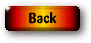Last revised: 5/12/2014